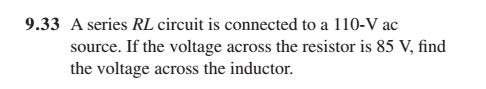# RL circuit Q. I must be missed something simple

• Engineering

## Homework Statement## The Attempt at a Solution

I would show my working, but honestly, I'd really just like a hint as to how to begin, because I've tried multiple different things and just gotten tangled up in multiple equations that are way more complicated looking than the problem itself...I must be missing something simple.

The chapter prior to it is using phasors for all these exercises.

NascentOxygen
Staff Emeritus
First, you draw the circuit and mark on the vo!tages, and label them. In AC circuits, voltages have a magnitude and a phase.

Then you show how they are added, using Pythagoras.

•kostoglotov
First, you draw the circuit and mark on the vo!tages, and label them. In AC circuits, voltages have a magnitude and a phase.

Then you show how they are added, using Pythagoras.

So the voltage across the inductor is just the square root of the source voltage squared minus the resistor voltage squared. That gets the right answer. But I don't fully understand why.

For a long time I was thinking that the 85 V drop on the R was measured at some particular time in the AC cycle, and that's why it wasn't simply a matter of the V over the inductor being 110 - 85.

So, is it because the voltage is leading the current on the inductor? Isn't this basically a violation of KVL? (I'm not saying it is, I'm saying that's what it looks like to me right now). Because 85 + 69.82 doesn't add up to 110. How can we have more total voltage drops in our loop than exist at our source at any given time?

edit: wait, when the V over the R is 85 the V over the L won't be 69.82 will it? 69.82 will be the maximum V drop over the L...?

Last edited:
NascentOxygen
Staff Emeritus
So the voltage across the inductor is just the square root of the source voltage squared minus the resistor voltage squared. That gets the right answer. But I don't fully understand why.
... the square root of the amplitude of the source vo!tage squared minus the amplitude of the resistor voltage squared,

where "amplitude" refers to the peak value of the sinusoidal waveform (you can also scale this to RMS values).

For a long time I was thinking that the 85 V drop on the R was measured at some particular time in the AC cycle, and that's why it wasn't simply a matter of the V over the inductor being 110 - 85.
By convention, the figure of 85 refers to [unless otherwise stated] the RMS value of the sinusoid; just as does the 110.

when the V over the R is 85 the V over the L won't be 69.82 will it? 69.82 will be the maximum V drop over the L...?
Right.

KVL holds at every instance. When vR(t)is momentarily at 85, then to this the algebraic addition of vL(t) at that instant will equal the value of the mains sinusoid at that instant.

•kostoglotov